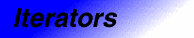# OutputIteratorCategory: iterators Component type: concept

## Description

An Output Iterator is a type that provides a mechanism for storing (but not necessarily accessing) a sequence of values. Output Iterators are in some sense the converse of InputIterator, but they have a far more restrictive interface: they do not necessarily support member access or equality, and they do not necessarily have either an associated distance type or even a value type . Intuitively, one picture of an Output Iterator is a tape: you can write a value to the current location and you can advance to the next location, but you cannot read values and you cannot back up or rewind.

None. 

## Notation

 `X` A type that is a model of Output Iterator `x`, `y` Object of type `X`

## Definitions

If `x` is an Output Iterator of type `X`, then the expression `*x = t;` stores the value `t` into `x`. Note that `operator=`, like other C++ functions, may be overloaded; it may, in fact, even be a template function. In general, then, `t` may be any of several different types. A type `T` belongs to the set of value types of `X` if, for an object `t` of type `T`, `*x = t;` is well-defined and does not require performing any non-trivial conversions on `t`. 

An Output Iterator may be singular, meaning that the results of most operations, including copying and dereference assignment, are undefined. The only operation that is guaranteed to be supported is assigning a nonsingular iterator to a singular iterator.

An Output Iterator may be dereferenceable, meaning that assignment through it is defined. Dereferenceable iterators are always nonsingular, but nonsingular iterators are not necessarily dereferenceable.

## Valid expressions

Name Expression Type requirements Return type
Default constructor
```X x;
X()
```

Copy constructor `X(x)`   `X`
Copy constructor `X y(x);` or `X y = x;`
Dereference assignment `*x = t` `t` is convertible to a type in the set of value types of `X`.  Result is not used
Preincrement `++x`   `X&`
Postincrement `(void) x++`   `void`
Postincrement and assign `*x++ = t;`   Result is not used

## Expression semantics

Name Expression Precondition Semantics Postcondition
Default constructor
```X x;
X()
```
`x` may be singular
Copy constructor `X(x)` `x` is nonsingular   `*X(x) = t` is equivalent to `*x = t` 
Copy constructor `X x(y);` or `X x = y;` `y` is nonsingular   `*y = t` is equivalent to `*x = t` 
Dereference assignment `*x = t` `x` is dereferenceable. If there has been a previous assignment through `x`, then there has been an intervening increment. 
Preincrement `++x` `x` is dereferenceable. `x` has previously been assigned through. If `x` has previously been incremented, then there has been an intervening assignment through `x`     `x` points to the next location into which a value may be stored
Postincrement `(void) x++` `x` is dereferenceable. `x` has previously been assigned through. Equivalent to `(void) ++x` `x` points to the next location into which a value may be stored
Postincrement and assign `*x++ = t;` `x` is dereferenceable. If there has been a previous assignment through `x`, then there has been an intervening increment.   Equivalent to `{*x = t; ++x; }` `x` points to the next location into which a value may be stored

## Complexity guarantees

The complexity of operations on output iterators is guaranteed to be amortized constant time.